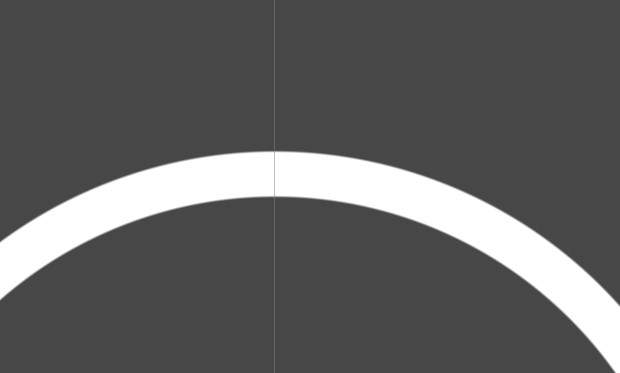# Shader shape function: draw a ring with alpha transparency

I am drawing a simple ring shape, with shaderlab code. I have innner and outer parameters and I just use a step function to switch the alpha from 0 to 1 and back to 0. This works but it’s very abrupt, how can I soften the edges? I’m thinking smooth step but I’m not sure how that works in this context.

``````fixed4 frag(v2f i) : SV_Target  {
//_Inner & _Outer are set in parameters

float alpha = step(_Inner, length(i.position.xy)) - step( _Outer, length(i.position.xy));

fixed3 finalColor = fixed3(_RingColor.rgb) ;
return fixed4(finalColor, alpha);
}
``````

To soften the edges of the ring, you can use the smoothstep function instead of the step function. The smoothstep function provides a smooth transition between two values, rather than a sudden switch as with the step function.

Here’s an example of how you could use the smoothstep function in your shader:

``````fixed4 frag(v2f i) : SV_Target  {
// _Inner and _Outer are set in parameters

// Calculate the alpha value using smoothstep
float alpha = smoothstep(_Inner, _Outer, length(i.position.xy));

fixed3 finalColor = fixed3(_RingColor.rgb) ;
return fixed4(finalColor, alpha);
}
``````

This will give you a smooth transition from full transparency to full opacity as the distance from the center of the ring increases.

omg prefect thank you! It doesn’t draw the ring inner & outer but I made some minor changes. Thanks !``````Shader "Unlit/circleShader"
{
Properties
{
_Inner("inner diameter", Range(0, 0.5)) = 0.05
_Outer("outer diameter", Range(0, 0.5)) = 0.5
_Soften("soften edge", Range(0, 0.05)) = 0.1
_RingColor("Ring color", COLOR) = (1, 1, 1, 1)
}
{
Tags { "RenderType"="Transparent" }
LOD 100
Blend SrcAlpha OneMinusSrcAlpha
Pass
{
CGPROGRAM
#pragma vertex vert
#pragma fragment frag
#include "UnityCG.cginc"

struct appdata
{
float4 vertex : POSITION;
float2 uv : TEXCOORD0;
};

struct v2f
{
float2 uv : TEXCOORD0;
float4 vertex : SV_POSITION;
float4 position: TEXCOORD1;
};

half _Outer;
half _Inner;
half _Soften;
float4 _RingColor;

v2f vert (appdata v)
{
v2f o;
o.vertex = UnityObjectToClipPos(v.vertex);
o.position = v.vertex;
return o;
}

fixed4 frag(v2f i) : SV_Target
{
// Calculate the alpha value using smoothstep
float alpha = smoothstep(_Inner, _Inner + _Soften, length(i.position.xy)) - smoothstep(_Outer, _Outer + _Soften, length(i.position.xy));
fixed3 finalColor = fixed3(_RingColor.rgb) ;
return fixed4(finalColor, alpha);
}
ENDCG
}
}
}
``````